# Parallel For in C#

## Parallel For Loop in C# with Examples

In this article, I am going to discuss the static Parallel For Loop in C# with Examples. Please read our previous article before proceeding to this article where we discussed the basic concepts of Parallel Programming in C#.  As part of this article, we will discuss the need and use of the Parallel For Loop compared with the C# standard For Loop.

##### Parallel For Loop in C#:

In this article, we will try to understand the parallel for loop in C#. We know that we can use a standard for loop in C# to iterate a block of code and this execution of the code will be done sequentially. For example, let’s say we want to print the numbers from 1 to 10 sequentially, then we can use a for loop as shown in the below example.

```using System;
namespace ParallelProgrammingDemo
{
class Program
{
static void Main(string[] args)
{
Console.WriteLine("C# For Loop");
for (int i = 1; i <= 10; i++)
{
Console.WriteLine(i);
}
}
}
}```
###### Output: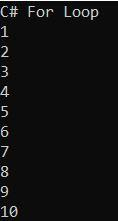But how did we know that the numbers were going to be printed in order from one to ten? Well, because our for loop executes sequentially. From the first one which is one until the last one which in this case is going to be the number ten. But what happens if we want to execute the different iterations of this block of code simultaneously. For that, we can use Parallel For Loop. With Parallel For Loop in C#, we have something very similar to the standard for loop but the main difference is that with Parallel For Loop the different executions are going to be done in parallel. The following example is the parallel version of the previous example.

```using System;

namespace ParallelProgrammingDemo
{
class Program
{
static void Main(string[] args)
{
Console.WriteLine("C# Parallel For Loop");

//It will start from 1 until 10
//Here 1 is the start index which is Inclusive
//Here 11 us the end index which is Exclusive
//Here number is similar to i of our standard for loop
//The value will be store in the variable number
Parallel.For(1, 11, number => {
Console.WriteLine(number);
});
}
}
}```

With Standard for loop, we can predict the order in which the numbers would appear on the console, but with Parallel For loop, we cannot do that. Now, run the application and see the output. Try to run the code multiple times and you might get different orders of the numbers in the console.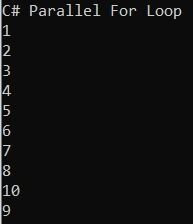##### What is Parallel For Loop in C#?

There are multiple overloaded versions of the Parallel For loop available in C#. In our example, we use the following overloaded versions.

1. public static ParallelLoopResult For(int fromInclusive, int toExclusive, Action<int> body): This method is used to execute a for loop in which iterations may run in parallel. Here, the parameter fromInclusive specifies the start index, inclusive. The parameter toExclusive specifies the end index, exclusive. And the parameter body specifies the delegate that is invoked once per iteration. It returns a structure that contains information about which portion of the loop is completed. It will throw ArgumentNullException if the body argument is null.

Note: For is a static method belongs to the Parallel static class. So, we need to invoke the method using the class name followed by the dot operator.

##### What is the difference between the Parallel For loop and Standard C# for loop?

The main difference between the Parallel For loop and the standard C# for loop is as follows

1. In the case of the standard C# for loop, the loop is going to run using a single thread whereas, in the case of the Parallel For loop, the loop is going to execute using multiple threads.
2. The second difference is that, in the case of the standard C# for loop, the loop is iterated in sequential order whereas, in the case of the Parallel For loop, the order of the iteration is not going to be in sequential order.

Note: When the iterations are independent of each other, it means that subsequent iterations do not need the state updates made by previous iterations, then in such cases, we need to use Task Parallel Library (TPL) to run each iteration in parallel on all the available cores. Moreover, the iteration should be an expensive iteration otherwise we will get negative performance, which we will also discuss as part of this article.

###### Syntax: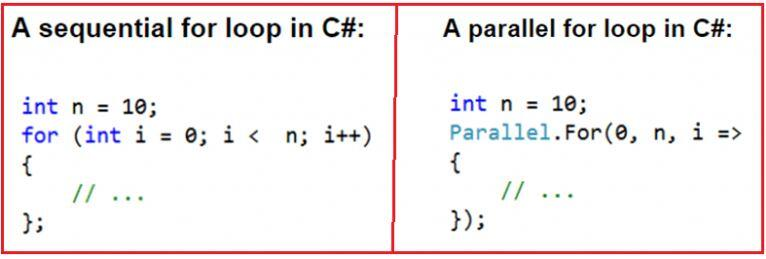###### Example to understand the differences between Standard For Loop and Parallel For Loop in C#:
```using System;

namespace ParallelProgrammingDemo
{
class Program
{
static void Main(string[] args)
{
Console.WriteLine("C# For Loop");
int number = 10;
for (int count = 0; count < number; count++)
{
//represents a unique identifier for the current managed thread.
//Sleep the loop for 10 miliseconds
}
Console.WriteLine();

Console.WriteLine("Parallel For Loop");
Parallel.For(0, number, count =>
{
//Sleep the loop for 10 miliseconds
});
}
}
}```

Once you run the above code, you will get the following output.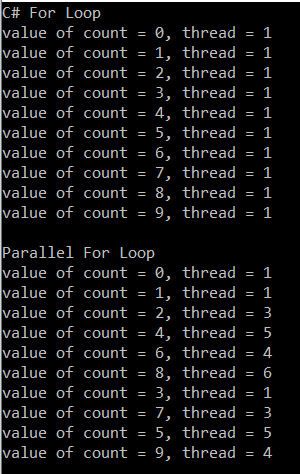As you can see in the above output, the standard C# for loop iterates sequentially using a single thread as a result, the results are printed sequentially. On the other hand, you can see with the Parallel for loop the results are not printed in sequential order. This is because it uses multiple threads to iterate over the collection. You can see that in our example it uses five threads to execute the code. It may vary in your system.

So this means that although we are guaranteed that the 10 executions will happen, we don’t know ahead of time the order of the executions of the iterations of the Parallel For Loop which means that if you have a block of code that you want to iterate over several times, if you want to speed things up and the operations can be done in any order, then you can consider using parallel For Loop in C#.

##### Example for a Better Understanding From a Performance Point of View.

First, we will write the example using C# for loop and will see how much time it will take to complete the execution. Then we will write the same example using the Parallel For method and will see how much time it will take to complete the execution.

In the below example, we create a sequential loop. The loop iterates ten times, and the loop control variable increases from zero to nine. In each iteration, the DoSomeIndependentTask method is called. The DoSomeIndependentTask method performs a calculation that is included to generate a long enough pause to see the performance improvement of the parallel version.

```using System;
using System.Diagnostics;

namespace ParallelProgrammingDemo
{
class Program
{
static void Main()
{
DateTime StartDateTime = DateTime.Now;
Stopwatch stopWatch = new Stopwatch();

Console.WriteLine("For Loop Execution start");
stopWatch.Start();
for (int i = 0; i < 10; i++)
{
Console.WriteLine("{0} - {1}", i, total);
}
DateTime EndDateTime = DateTime.Now;
Console.WriteLine("For Loop Execution end ");
stopWatch.Stop();
Console.WriteLine(\$"Time Taken to Execute the For Loop in miliseconds {stopWatch.ElapsedMilliseconds}");

}

{
//Do Some Time Consuming Task here
//Most Probably some calculation or DB related activity
long total = 0;
for (int i = 1; i < 100000000; i++)
{
total += i;
}
}
}
}```
###### Output: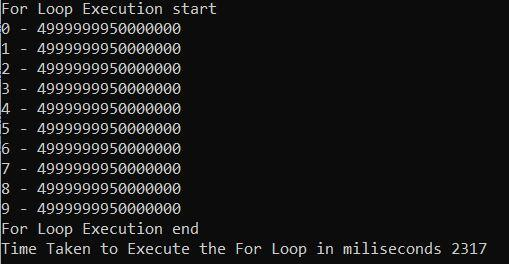As you can see from the above output the for loop statement took approximately 2317 milliseconds to complete the execution. In your machine, the time may vary. Now, do one thing. While running the code, open Task Manager and observe the CPU Utilization. In my machine maximum of 42%, CPU Utilization is done as shown in the below image. You just need to run the code and at the same time, you need to observe the CPU Utilization and see what is the maximum CPU Utilization is done on your machine.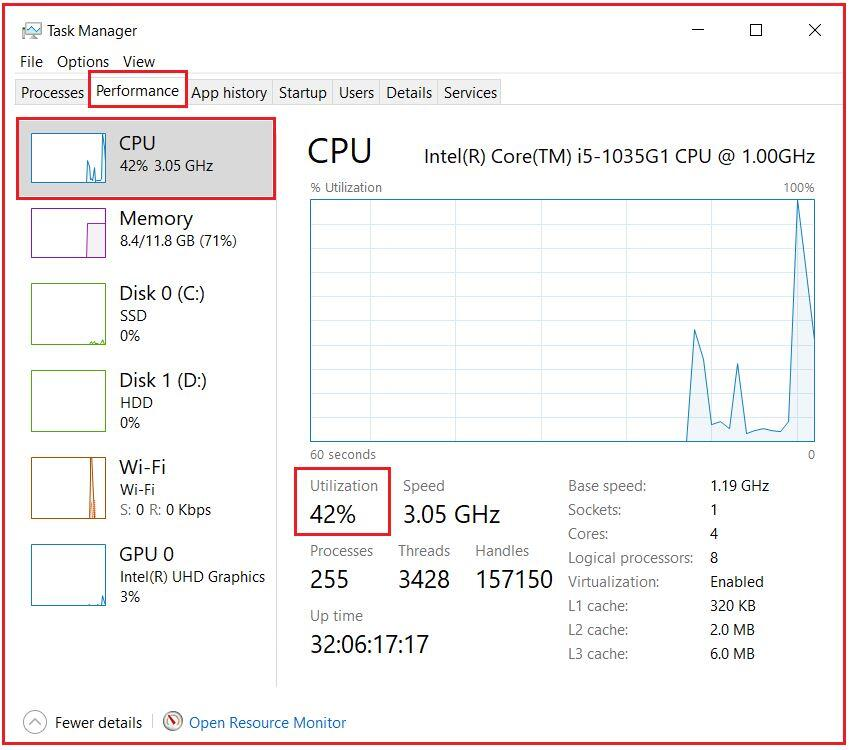In the above example, the DoSomeIndependentTask method does not call any external services or APIs so it is a CPU Bound operation. The CPU-bound operations are those operations whose resolution depends on the processor, not on services external to the application. In general, we need to use parallelism in CPU-bound operations, Let’s rewrite the same example using the Parallel For method and see the performance.

```using System;
using System.Diagnostics;

namespace ParallelProgrammingDemo
{
class Program
{
static void Main()
{
DateTime StartDateTime = DateTime.Now;
Stopwatch stopWatch = new Stopwatch();

Console.WriteLine("Parallel For Loop Execution start");
stopWatch.Start();

Parallel.For(0, 10, i => {
Console.WriteLine("{0} - {1}", i, total);
});

DateTime EndDateTime = DateTime.Now;
Console.WriteLine("Parallel For Loop Execution end ");
stopWatch.Stop();
Console.WriteLine(\$"Time Taken to Execute Parallel For Loop in miliseconds {stopWatch.ElapsedMilliseconds}");

}

{
//Do Some Time Consuming Task here
//Most Probably some calculation or DB related activity
long total = 0;
for (int i = 1; i < 100000000; i++)
{
total += i;
}
}
}
}```
###### Output: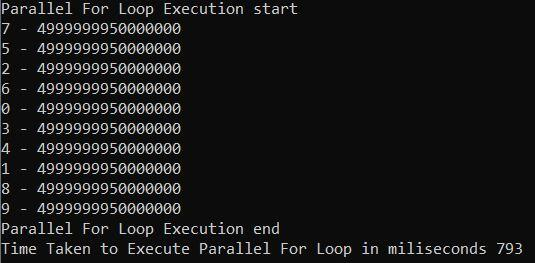As shown in the above output, the Parallel For method took 793 milliseconds to complete the execution as compared with the 2317 milliseconds with the standard for loop execution. Again, do the same thing. While running the code, open Task Manager and observe the CPU Utilization. In my machine maximum of 100%, CPU Utilization is done at some point of time while running the code as shown in the below image. You just need to run the code and at the same time, you need to observe the CPU Utilization and see what is the maximum CPU Utilization is done on your machine.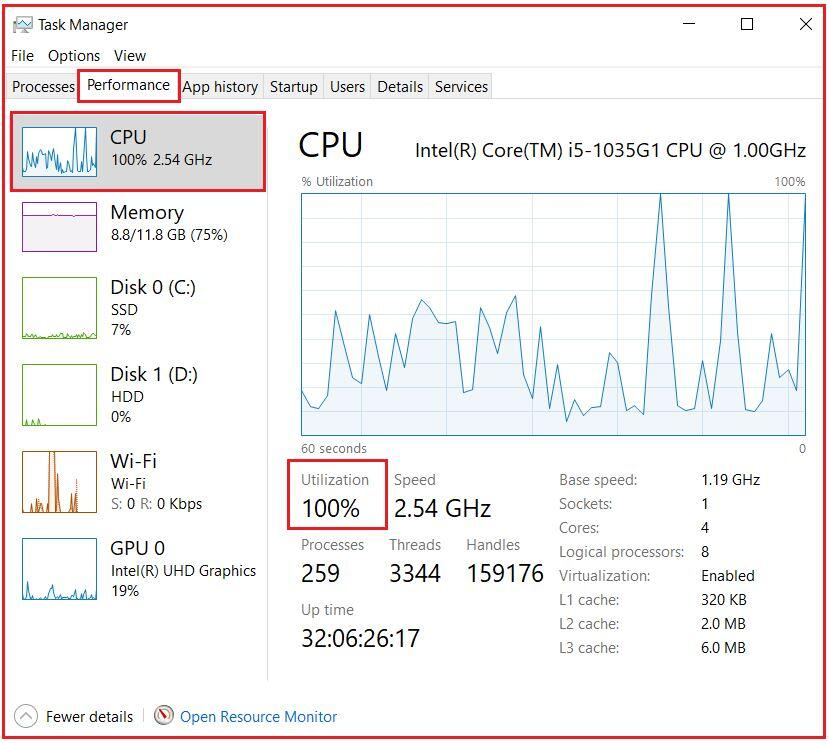So, the parallel version of the For loop gives you better performance as compared with the standard for loop. But this is not always true. Sometimes the standard for loop will give you better performance compared to the Parallel For loop which we will discuss in our upcoming article.

##### ParallelOptions Class in C#

The ParallelOptions class is one of the most useful classes when working with multithreading. This class provides options to limit the number of concurrently executing loop methods.

##### The Degree of Parallelism in C#:

Using the Degree of parallelism we can specify the maximum number of threads to be used to execute the program. Following is the syntax to use ParallelOptions class with a Degree of Parallelism.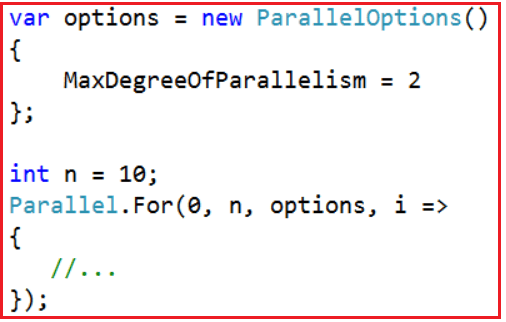The MaxDegreeOfParallelism property affects the number of concurrent operations run by Parallel method calls that are passed this ParallelOptions instance. A positive property value limits the number of concurrent operations to the set value. If it is -1, there is no limit on the number of concurrently running operations.

##### Example to Understand MaxDegreeOfParallelism in C#
```using System;

namespace ParallelProgrammingDemo
{
class Program
{
static void Main(string[] args)
{
//Limiting the maximum degree of parallelism to 2
var options = new ParallelOptions()
{
MaxDegreeOfParallelism = 2
};
int n = 10;
Parallel.For(0, n, options, i =>
{
Console.WriteLine(@"value of i = {0}, thread = {1}",
});
Console.WriteLine("Press any key to exist");
}
}
}```
###### Output: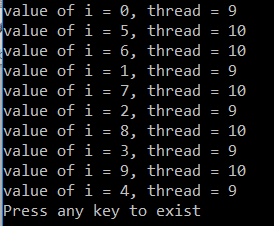As we set the degree of parallelism to 2. So, a maximum of 2 threads is used to execute the code that we can see from the above output.

##### Terminating a Parallel For Loop in C#:

The below example shows how to break out of a For loop and also how to stop a loop. In this context, “break” means completing all iterations on all threads that are prior to the current iteration on the current thread, and then exiting the loop. “Stop” means to stop all iterations as soon as convenient.

```using System;
using System.Linq;

namespace ParallelProgrammingDemo
{
class Program
{
static void Main()
{
var BreakSource = Enumerable.Range(0, 1000).ToList();
int BreakData = 0;
Console.WriteLine("Using loopstate Break Method");
Parallel.For(0, BreakSource.Count, (i, BreakLoopState) =>
{
BreakData += i;
if (BreakData > 100)
{
BreakLoopState.Break();
Console.WriteLine("Break called iteration {0}. data = {1} ", i, BreakData);
}
});
Console.WriteLine("Break called data = {0} ", BreakData);

var StopSource = Enumerable.Range(0, 1000).ToList();
int StopData = 0;
Console.WriteLine("Using loopstate Stop Method");
Parallel.For(0, StopSource.Count, (i, StopLoopState) =>
{
StopData += i;
if (StopData > 100)
{
StopLoopState.Stop();
Console.WriteLine("Stop called iteration {0}. data = {1} ", i, StopData);
}
});

Console.WriteLine("Stop called data = {0} ", StopData);
}
}
}
```
###### Output: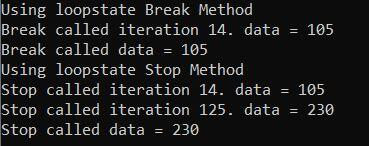In Parallel.For or Parallel.ForEach Loop in C#, you cannot use the same break or Exit statement that is used in a sequential loop because those language constructs are valid for loops, and a parallel “loop” is actually a method, not a loop. Instead, you use either the Stop or Break method.

In the next article, I am going to discuss the Parallel ForEach Method in C# with some examples. Here, In this article, I try to explain the Parallel For in C# with some examples. I hope you understood the need and use of the Parallel For method in C#.

### 3 thoughts on “Parallel For in C#”

1.1.2.